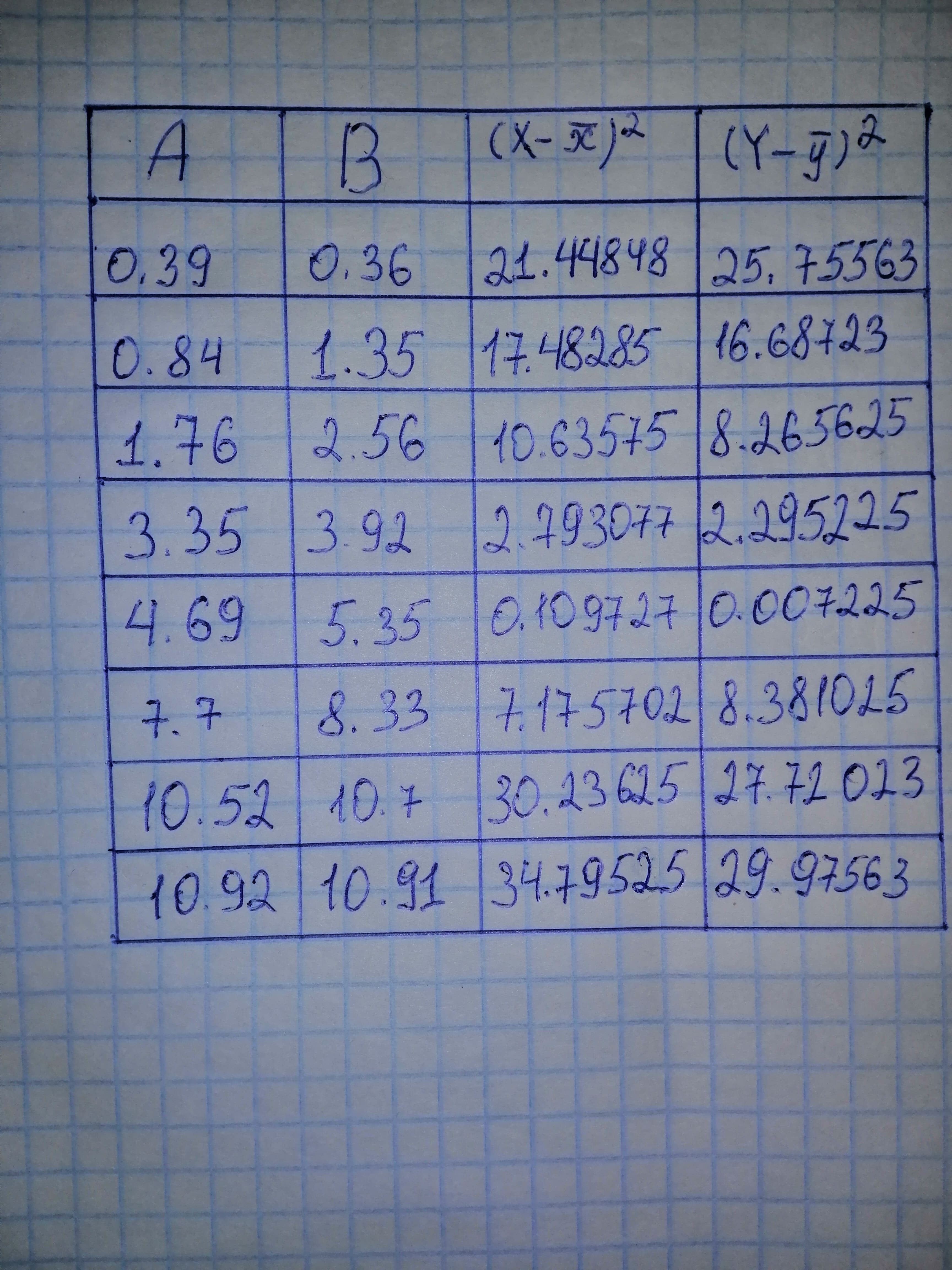# Two different analytical methods were used to determine residual chlorine in sewage effluents. Both methods were used on the same samples, but each caRamsey 2021-01-06 Answered
Two different analytical methods were used to determine residual chlorine in sewage effluents. Both methods were used on the same samples, but each came from various locations, with differing amounts of contact time with the effluent. The concentration of Cl in mg//L was determined by the two methods, and the following results were obtained:
Method $A:0.39,0.84,1.76,3.35,4.69,7.70,10.52,10.92$
Method $B:0.36,1.35,2.56,3.92,5.35,8.33,10.70,10.91$
(a) What type of t test should be used to compare the two methods and why?
(b) Do the two methods give different results?
(c) Does the conclusion depend on whether the 90%, 95% or 99% confidence levels are used?
You can still ask an expert for help

• Questions are typically answered in as fast as 30 minutes

Solve your problem for the price of one coffee

• Math expert for every subject
• Pay only if we can solve itRoosevelt Houghton

Step 1The mean is given by
$\stackrel{―}{x}=\frac{1}{n}\sum x$
$A\right)\stackrel{―}{x}=5.02125$
$B\right)\stackrel{―}{y}=5.435$
The standard deviation is given by
$\sigma =\frac{\sqrt{1}}{n-1}\sum \left(x-\stackrel{―}{x}{\right)}^{2}$
$A\right){s}_{1}=4.2203$
$B\right){s}_{1}=4.1246$
Step 2
a) We will be using an independent two-sample t-test as we are comparing two different populations.
b) The null and alternative hypotheses are
${H}_{0}:{u}_{1}={u}_{2}$
${H}_{1}:{u}_{1}\ne {u}_{2}$
The test statistic is given by
$t=\frac{\stackrel{―}{x}-\stackrel{―}{y}}{\sqrt{\frac{{s}_{1}^{2}}{{n}_{1}}+\frac{{s}_{2}^{2}}{{n}_{2}}}}$
$t=\frac{5.02125-5.435}{\sqrt{\frac{\left(4.2203{\right)}^{2}}{8}+\frac{\left(4.1246{\right)}^{2}}{8}}}$
$t=-0.19831$
p - value $=0.8446$
Since the p-value is greater than 0.05, we fail to reject the null hypothesis and conclude that there is no significant difference between the two methods.